# Shell Scripting – Functions and it’s types

• Last Updated : 03 Jun, 2022

A shell is a command-line interpreter, and shell scripts commonly execute file manipulation, program execution, and text output. In this article, we are going to read about functions and their types in shell scripting.

## Function

A function is a collection of statements that execute a specified task. Its main goal is to break down a complicated procedure into simpler subroutines that can subsequently be used to accomplish the more complex routine.For the following reasons, functions are popular:

• Assist with code reuse.
• Enhance the program’s readability.
• Modularize the software.
• Allow for easy maintenance.

The basic structure of a function in shell scripting looks as follows:

```function_name(){
// body of the function
}```

The function_name can be any valid string and the body can be any sequence of valid statements in the scripting language.

Let us try to understand the concept of functions by looking at an example. The following is a code to print all the prime numbers between a range [le,ri]. It consists of a function is_prime() which is used to check if the given number is a prime or not. In this function, we use the variable \$1 to access the first argument, which is the number itself. In scripting languages, we can access arguments by \$i, where i is a number that signifies the position of the argument.

```echo -n "Enter Left-End: "
echo -n "Enter Right-End: "

is_prime(){
if [ \$1 -lt 2 ]; then
return
fi
ctr=0
for((i=2;i<\$1;i++)){
if [ \$(( \$1 % i )) -eq 0 ]; then
ctr=\$(( ctr +1 ))
fi
}
if [ \$ctr -eq 0 ]; then
printf "%d " "\$1"
fi
}
printf "Prime Numbers between %d and %d are: " "\$le" "\$ri"
for((i=le;i<=ri;i++)){
is_prime \$i
}
printf "\n" ```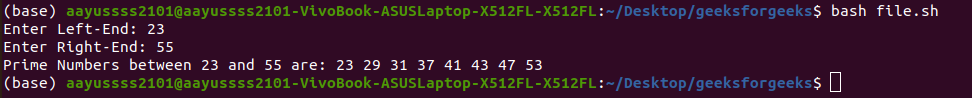## Types of Functions

The functions in shell scripting can be boxed into a number of categories. The following are some of them:

1. The functions that return a value to the caller. The return keyword is used by the functions for this purpose.

The following is one such function used to calculate the average of the given numbers.

```find_avg(){
len=\$#
sum=0
for x in "\$@"
do
sum=\$((sum + x))
done
avg=\$((sum/len))
return \$avg
}
find_avg 30 40 50 60
printf "%f" "\$?"
printf "\n"```

Output: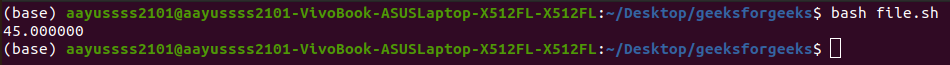Remember that return can only return a number (0-255).

2. The functions that terminate the shell using the exit keyword.

```is_odd(){
x=\$1
if [ \$((x%2)) == 0 ]; then
echo "Invalid Input"
exit 1
else
echo "Number is Odd"
fi
}
is_odd 64```

Output: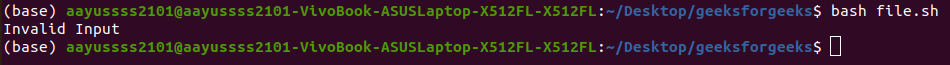3. The functions that alter the value of a variable or variables.

```a=1
increment(){
a=\$((a+1))
return
}
increment
echo "\$a"```

Output: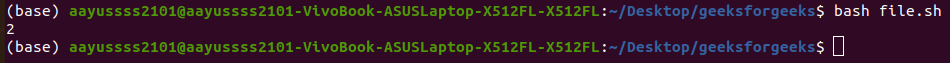4. The functions that echo output to the standard output.

```hello_world(){
echo "Hello World"
return
}
hello_world```

Output: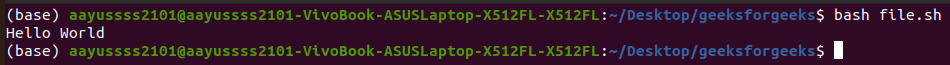My Personal Notes arrow_drop_up